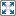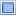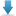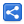# Java Regex - Greedy Quantifiers

[Last Updated: Feb 9, 2019]

A regex quantifier tells the regex engine to match a character or group of characters for specified number of times . For example the regex `X+` will cause the engine to match one or more Xs.

By default (as in example X+) Java regex quantifiers are greedy, the regex engine starts out by matching as many characters it can. We can change this default behavior by appending another metacharacter e.g. X+?. In this tutorial we are going to cover default quantifiers `+`, `*`, `?` and `{ }`.

Let's see an example to see the how a default greedy quantifier works.

```  public static void main(String[] args) {
match("X", "XXX");
match("X+", "XXX");
}

public static void match(String regex, String input) {
Pattern pattern = Pattern.compile(regex);
Matcher matcher = pattern.matcher(input);

while (matcher.find()) {
String matchedValue = matcher.group();
System.out.printf("Matched startIndex= %s, endIndex= %s, match: '%s'\n",
matcher.start(), matcher.end(), matchedValue);
}

System.out.println("-------------------");
}```

Output:

```Matched startIndex= 0, endIndex= 1, match: 'X'
Matched startIndex= 1, endIndex= 2, match: 'X'
Matched startIndex= 2, endIndex= 3, match: 'X'
-------------------
Matched startIndex= 0, endIndex= 3, match: 'XXX'
-------------------
```

Comparing the two outputs, we can see the behavior of the greedy '+' quantifier. Let's explore more details of different type of quantifier and their behavior in different scenarios.

Regex Construct/Terms Examples
`+` The plus Greedy Quantifier:
It matches one or more times.

How Greedy Quantifier works?

Depending on the provided pattern, regex engine tries to read/eat the input string as much as possible before attempting for a match. Let's understand that with examples.

Consider the pattern ".+" (matches any character but line terminator, one or more times) and input string "abx".
Followings are the steps the engines goes through:

1. The engine tries to eat the longest part of the input string, satisfying the pattern ".+". As a result the entire input string "abx" is eaten by the engine because it completely satisfies the pattern ".+"
2. Now engine will try to make a match. Since there's only greedy part in the pattern and nothing else. The whole eaten string is a match. That's it.

Now consider the pattern ".+x" (matches any character other than line terminator, one or more times, followed by literal x) and the input string "abx".
Followings are the step.

1. There are two parts of the regex pattern ".+" and "x". The engine will start with the first part i.e. ".+"
2. Since the entire input string "abx" satisfying the first part of the pattern ".+", it is eaten by the engine.
3. Now the engine will look at the other part of the pattern i.e. the literal "x". As the entire input string has been eaten by the engine, there's nothing left for "x" to match.
4. Now rather than admitting failure, the engine at this point, will give up one character from the eaten input string. It will do that from the right end of the string. This process is also known as backing off or backtracking by one character.
5. Now the eaten string becomes "ab" which of course still satisfies the pattern ".+"
6. The given up character "x" exactly matches the pattern's literal part "x"
7. Finally both parts of the pattern ".+x" are satisfied, hence we have a single match of entire string.

Now consider the pattern "x+" (matches one or more x) and the input string "xxbxxx".
Now let's see what happens here:

1. There's only one part of regex pattern i.e. "x+". Now engine will look at the input string to eat the longest part satisfying the pattern "x++".
2. Since the entire string is not satisfying that because of the presence of a "b", the engine will look at the shorter regions/parts but will still try to eat the longest lengths in those regions.
3. Looking at the pattern "x+" again, the two parts "xx" and "xxx" and are separately satisfying the pattern "x+" and no backtracking is needed for each part (that's out of question here anyways because x+ can never eat characters which are not x, but .+ can).
4. Finally we have two matches. We have to call `Mather#find` twice to have two matches separately.

#### Quantifying the matches

Generally speaking, in all above scenarios and regardless of, we have greedy or some other type of quantifier. The very last thing engine will do, is to look at the associated defined length/quantity with quantifier to possibly break down the match into multiple matches.
To understand that consider our very last example of pattern "x+" and input "xxbxxx" where we had two matches "xx" and "xxx". The first and second, both parts won't get broken down quantitatively, because they both satisfy the quantifier "+" criteria of "matches one or more times".
In case if some quantifier allows exactly one length match (as we will see the {} quantifier later) both matches will be broken down to individual results. So one match "xx" will become two matches of "x" and "x".

```/* This quantifier doesn't match zero-length input*/
Pattern.matches("a+", ""); //false
Pattern.matches(".+", ""); //false
Pattern.compile("a+")       .matcher("ababaaaab")       .find();//matches:  'a' at 0-1, 'a' at 2-3, 'aaaa' at 4-8
//'ababaaaab'

/* The first 'b' in the input string doesn't match because there has to be at least one 'a' before 'b', that's the definition of '+' quantifier*/
Pattern.compile("a+b")       .matcher("bbbaaab")       .find();//matches:  'aaab' at 3-7
//'bbbaaab'

/* The first 'ab' matches. Compare the last example.*/
Pattern.compile("a+b")       .matcher("abbbaaab")       .find();//matches:  'ab' at 0-2, 'aaab' at 4-8
//'abbbaaab'

/* In this example the regex part 'a.*' eats the entire input 'axyb', and then looking at last literal part 'b' (nothing left for a match) it backtracks and give up the last eaten character b. Please see the left column for more examples.*/
Pattern.compile("a.+b")       .matcher("axyb")       .find();//matches:  'axyb' at 0-4
//'axyb'

/* In this example the entire input string is not satisfying 'a[.\\S]' because this part is expecting 'a' at the left followed by any characters but not white-space. In this case, the input string will be divided into two parts 'aaaxb' and 'azzzeb' as both are satisfying 'a[.\\S]'. Both will be eaten entirely by 'a[.\\S]' then backtracking will happen in both to match remaining part 'b' of the expression.*/
Pattern.compile("a[.\\S]+b")       .matcher("aaaxb azzzeb")       .find();//matches:  'aaaxb' at 0-5, 'azzzeb' at 6-12
//'aaaxb azzzeb'

Pattern.compile(".+x")       .matcher("abc x")       .find();//matches:  'abc x' at 0-5
//'abc x'

Pattern.compile("a+bx")       .matcher("aaabx")       .find();//matches:  'aaabx' at 0-5
//'aaabx'

Pattern.compile("x+")       .matcher("xxbxxx")       .find();//matches:  'xx' at 0-2, 'xxx' at 3-6
//'xxbxxx'

/* Note there's no greedy eating here too. The pattern must start */
Pattern.compile("A.+")       .matcher("AEG")       .find();//matches:  'AEG' at 0-3
//'AEG'

/* Matches xyz as one entity, one ore more times*/
Pattern.compile("(xyz)+")       .matcher("zz xyz abc xyzxyz xy")       .find();//matches:  'xyz' at 3-6, 'xyzxyz' at 11-17
//'zz xyz abc xyzxyz xy'

Pattern.compile("(xyz)+")       .matcher("zz xyz abc xyzxyz xy")       .replaceAll("S");//result: 'zz S abc S xy'

/* Either a or b, one or more times*/
Pattern.compile("(a|b)+")       .matcher("aaaa bb cabc")       .find();//matches:  'aaaa' at 0-4, 'bb' at 5-7, 'ab' at 9-11
//'aaaa bb cabc'

/* 'abcxyz' and 'xyzabc' are expectedly matched but why matched together instead of separately? That's because it's the greedy quantifier, the regex engine tries to match as many characters it can in one attempt.*/
Pattern.compile("((abc)|(xyz))+")       .matcher("abc xyz zyxabc abcxyz xyzabc")       .find();//matches:  'abc' at 0-3, 'xyz' at 4-7, 'abc' at 11-14,                //'abcxyz' at 15-21, 'xyzabc' at 22-28
//'abc xyz zyxabc abcxyz xyzabc'

Pattern.compile("[0-9]+")       .matcher("9823 340")       .find();//matches:  '9823' at 0-4, '340' at 5-8
//'9823 340'

Pattern.compile("([0-9]|\\W)+")       .matcher("9823 340")       .find();//matches:  '9823 340' at 0-8
//'9823 340'

/* Greedy quantifier picks the longest match.*/
Pattern.compile(".+ten")       .matcher("AtenAAAAAten")       .find();//matches:  'AtenAAAAAten' at 0-12
//'AtenAAAAAten'

Pattern.compile("A.+ten")       .matcher("xtenAAAAAten")       .find();//matches:  'AAAAAten' at 4-12
//'xtenAAAAAten'

Pattern.compile("ten+")       .matcher("tenAAAAAten")       .find();//matches:  'ten' at 0-3, 'ten' at 8-11
//'tenAAAAAten'

/* Matching a file extension*/
Pattern.compile("(\\.[^.]+)\$")       .matcher("abc.xyz.appp.version.exe")       .find();//matches:  '.exe' at 20-24
//'abc.xyz.appp.version.exe'

```
`*` The Asterisk (star) Greedy Quantifier:
It matches zero or more times.

Important points:
• As opposed to plus quantifier in the last section, this quantifier can match an input string or it's parts, zero number of times. That means anything in the input string that's not a "match" at a particular attempt will show up as a zero-length match. This rule doesn't apply if the regex pattern as a whole doesn't allow zero length e.g. "XY*" (the quantifier metacharacter * is only with Y and not with X, so Y* can be seen as empty but not X)
• Methods Matcher#start() and Matcher#end() both returns the same indexes at zero-length matches. Matcher#group() returns empty string.
• For pattern of type .* or X*, we will have zero length match at the end of the input string too. That's because at the end of a string we have nothing and the pattern .* or X* can accept nothing at the right. On the other hand the pattern "A*X" or or X.*", ".*X" won't match at the end because non of them (as a whole) matches zero length (empty) string.
```/* Matches empty input because this quantifier allows zero-length match.*/
Pattern.matches(".*", ""); //true
Pattern.compile(".*")       .matcher("")       .find();//matches:  '' at 0-0

Pattern.matches(".*", "Anything matches"); //true

/* Notice zero-length match at the end.*/
Pattern.compile(".*")       .matcher("Anything matches")       .find();//matches:  'Anything matches' at 0-16, '' at 16-16
//'Anything matches'

Pattern.compile(".*")       .matcher("Anything matches")       .replaceAll("S T");//result: 'S TS T'

Pattern.matches(".*x", "Sandbox"); //true

/* Notice no zero-length result here.*/
Pattern.compile(".*x")       .matcher("Sandbox")       .find();//matches:  'Sandbox' at 0-7
//'Sandbox'

Pattern.compile("x.*")       .matcher("xenon")       .find();//matches:  'xenon' at 0-5
//'xenon'

Pattern.matches(".*test", "testyyxxtest"); //true

/* Greedy behavior*/
Pattern.compile(".*test")       .matcher("testyyxxtest")       .find();//matches:  'testyyxxtest' at 0-12
//'testyyxxtest'

/* The first white space is the match for '\\W' part. Also notice there's no zero-length match at the end, because '\\W.*' doesn't match empty or zero-length string.*/
Pattern.compile("\\W.*")       .matcher("this is a sentence")       .find();//matches:  ' is a sentence' at 4-18
//'this is a sentence'

/* Notice zero-length matches with each attempt. The pattern 't*' can accept empty string*/
Pattern.compile("t*")       .matcher("yx")       .find();//matches:  '' at 0-0, '' at 1-1, '' at 2-2

/* The reason we don't have zero-length match for each y sitting at the end of the input string is that: the engine can see '*a' as empty but expects exact 'bc' given by the whole pattern '*abc'*/
Pattern.compile(".*abc")       .matcher("zzzzabcyyy")       .find();//matches:  'zzzzabc' at 0-7
//'zzzzabcyyy'

Pattern.compile("s*")       .matcher("ssssssst")       .find();//matches:  'sssssss' at 0-7, '' at 7-7, '' at 8-8
//'ssssssst'

/* In this replaceAll example the 'XY ' is placed exactly at the positions given by the matched indexes shown in the last example. In the result, there's also a 't' at the end, because t is not a match and cannot be replaced.*/
Pattern.compile("s*")       .matcher("ssssssst")       .replaceAll("XY ");//result: 'XY XY tXY '

Pattern.compile("l.*c")       .matcher("appeal to logic")       .find();//matches:  'l to logic' at 5-15
//'appeal to logic'

Pattern.compile("\\bl.*c\\b")       .matcher("appeal to logic")       .find();//matches:  'logic' at 10-15
//'appeal to logic'

/* Matching Even numbers*/
Pattern.compile("\\d*")       .matcher("3 46 33 88 133")       .find();//matches:  '3' at 0-1, '33' at 5-7, '133' at 11-14
//'3 46 33 88 133'

/* Matching HTML tag without attributes*/
Pattern.compile("<[A-Za-z][A-Za-z0-9]*>")       .matcher("<span>")       .find();//matches:  '<span>' at 0-6
//'<span>'

```
`?` The Question Mark Greedy Quantifier:

It matches once or not at all.

The ? quantifier matches the preceding element zero or exactly one time and not more than that. As it can match zero time too, we would see zero-length result in our examples, like we saw with `*` in the last section.

Though the ? is categorized as greedy quantifier, it's not greedy greedy (Not typo, I'm using lexical stress here). By it's basic definition of "matches once or not at all", we will not see this quantifier as greedy as we saw in the previous examples.

```/* It allows zero-length matches*/
Pattern.compile(".?")       .matcher("")       .find();//matches:  '' at 0-0

/* It doesn't allow more than one match. That means it will not match 'aaaa' together*/
Pattern.compile(".?")       .matcher("aaaa")       .find();//matches:  'a' at 0-1, 'a' at 1-2, 'a' at 2-3, 'a' at 3-4,                //'' at 4-4
//'aaaa'

/* Notice zero length match for b and at the end*/
Pattern.compile("a?")       .matcher("aab")       .find();//matches:  'a' at 0-1, 'a' at 1-2, '' at 2-2, '' at 3-3
//'aab'

Pattern.compile("a?")       .matcher("aab")       .replaceAll("X-");//result: 'X-X-X-bX-'

Pattern.compile(".?")       .matcher("red car")       .find();//matches:  'r' at 0-1, 'e' at 1-2, 'd' at 2-3, ' ' at 3-4,                //'c' at 4-5, 'a' at 5-6, 'r' at 6-7, '' at 7-7
//'red car'

Pattern.compile("(red)?")       .matcher("red car")       .find();//matches:  'red' at 0-3, '' at 3-3, '' at 4-4, '' at 5-5,                //'' at 6-6, '' at 7-7
//'red car'

Pattern.compile("([0-9][0-9][A-Z])?")       .matcher("89C34D555")       .find();//matches:  '89C' at 0-3, '34D' at 3-6, '' at 6-6, '' at 7-7,                //'' at 8-8, '' at 9-9
//'89C34D555'

Pattern.compile("wall(paper)?")       .matcher("wall wallpaper paper")       .find();//matches:  'wall' at 0-4, 'wallpaper' at 5-14
//'wall wallpaper paper'

```
`{ }` The Curly Brackets Greedy Quantifier:

Using this quantifier, we define min and/or max number of matches instead of above default number of times matches. Followings are the various ways we can do that.

 X{n} matches X, exactly n number of times. X{n,} matches X, at least n number of times. X{n,m} matches X, at least n number of times but not more than m times.

Followings are equivalents of the quantifiers we have discussed so far.

 + {1,} * {0,} ? {0,1}
```Pattern.compile("x{2}")       .matcher("xx xxx")       .find();//matches:  'xx' at 0-2, 'xx' at 3-5
//'xx xxx'

Pattern.compile("x{2,}")       .matcher("xx xxx xxxx")       .find();//matches:  'xx' at 0-2, 'xxx' at 3-6, 'xxxx' at 7-11
//'xx xxx xxxx'

Pattern.compile("x{1,3}")       .matcher("xx xxx xxxx")       .find();//matches:  'xx' at 0-2, 'xxx' at 3-6, 'xxx' at 7-10,                //'x' at 10-11
//'xx xxx xxxx'

/* This allows zero-length match*/
Pattern.compile("x{0,2}")       .matcher("xx xxx xxxx")       .find();//matches:  'xx' at 0-2, '' at 2-2, 'xx' at 3-5, 'x' at 5-6,                //'' at 6-6, 'xx' at 7-9, 'xx' at 9-11, '' at 11-11
//'xx xxx xxxx'

Pattern.compile("(li){2,}")       .matcher("lilies milliliter")       .find();//matches:  'lili' at 0-4, 'lili' at 10-14
//'lilies milliliter'

/* Words with three letters*/
Pattern.compile("\\b[a-z]{3,3}\\b")       .matcher("A fly has big eyes")       .find();//matches:  'fly' at 2-5, 'has' at 6-9, 'big' at 10-13
//'A fly has big eyes'

Pattern.compile("[a-z][0-9]{2,3}")       .matcher("aa29 a3333 3d43")       .find();//matches:  'a29' at 1-4, 'a333' at 5-9, 'd43' at 12-15
//'aa29 a3333 3d43'

Pattern.compile("([a-z][0-9]){2,2}")       .matcher("aa29 a3d4a3")       .find();//matches:  'a3d4' at 5-9
//'aa29 a3d4a3'

/* This matches one of the Math methods of length 3. */
Pattern.compile("Math[.](\\w){3,3}[(][^)(]{1,}[)];")       .matcher("Math.sin(20);  Math.abs(4);")       .find();//matches:  'Math.sin(20);' at 0-13, 'Math.abs(4);' at 15-27
//'Math.sin(20);  Math.abs(4);'

```

#### Example Project

Dependencies and Technologies Used:

• JDK 1.8
• Maven 3.0.4

 Regex Quantifier ExamplesSelect AllDownload• regex-quantifiers
• src
• main
• java
• com
• logicbig
• example
• RegexExamples.java

 Share